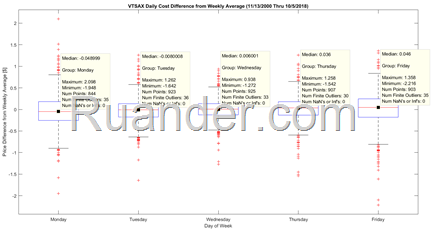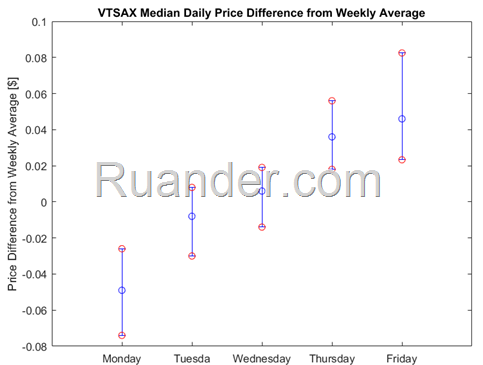## Pages

### Monte Carlo: Roll 2 Dice

Sometime ago I posted about the Sum of Two Dice. In that post, I explained the math behind it and I also performed a Monte Carlo Simulation in R. I would like to go back to this problem to show how my free Monte Carlo Simulation Excel file can be used in this situation.

Assuming a fair die, each die has six different possible output values (1, 2, 3, 4, 5, and 6) and the probability of any of these values should be equal (1/6). The probability function that describes this behavior is the uniform discrete probability function with a lower bound of 1 and an upper bound of 6. The reason that this is a discrete probability is that in between values that are not integers (such as 1.5) are not an option.

Using my Monte Carlo Simulation Excel file to simulate the sum of two dice is very straight forward. First, click "0. Reset"  in the Simulator sheet to make sure you are starting with a fresh and clean sheet. Indicate that the number of inputs is 2 by typing this value in cell F5. Remember, each input will represent one die. Now, click on "1. Define Inputs" and select the Uniform_Discrete probability function for both inputs with 1 as the lower bound (parameter 1) and 6 as the upper bound (parameter 2). Leave the other parameters empty. Your Simulator sheet should look like this:
Now indicate the number of simulations to perform by typing the number of simulations in cell F6. For this example, I will perform 1 million simulations knowing that this large number of simulations will likely take some time to complete so I will have to be patient. It took ~30 mins in a very slow computer on battery. Note that if I would have done 100K simulations it would have only taken about 3 minutes (time is approximately linear with number of simulations). Faster computers will finish faster.

Once the simulation is completed, there are a couple of things to do. First, in the "Y=" column, you will want to type the equation which is basically the sum of die 1 and die 2. This should look like this:

First, let's explore the output of each die. My Monte Carlo Simulation Excel file automatically outputs a histogram of your X1 variable if you have excel 2016 or higher. However, for discrete outcomes, I like making my own using the Excel Function COUNTIF. Doing this I obtained very similar outputs for both X1 and X2. Below I show the output for X1. Note that the number of occurrences for each number is about 1/6 of the total as expected.

In the same way, we can plot the histogram of our Y= X1 + X2. Note that in accordance with my previous post, the number 7 occurred approximately 16.6667% of the time. It is pretty interesting how the sum of two variables that have a uniform discrete probability function results in an output variable with a discrete triangular distribution function.

Now that we have looked into the sum of 2 dice, how about the sum of 3 dice, or 7 dice? Can you use my Monte Carlo Simulation Excel file to do this with more than 2 dice?

### Monte Carlo Simulation Excel

If you are looking for a FREE excel calculator to perform Monte Carlo Simulations you have arrived at the right place. Just download my FREE Excel Monte Carlo Simulator and start doing Monte Carlo simulations right in your already familiar Excel Environment.  I will provide a short intro below, but feel free to download my Excel Monte Carlo Simulator to get started right away.

If you are searching the Word Wide Web for “Monte Carlo Excel” you probably already know or have a general idea of how Monte Carlo Simulations work. The user typically has one or more variables with known probability distributions as well as a transfer function (basically an equation) to translate these input variables into the desired output variable. The goal in a Monte Carlo Simulation is to randomly retrieve a value for each of the input variables according to their probability distribution. Do this over and over multiple times (1k, 100k, 500k, 1 million times, and so on) and you can now understand what your output distribution (and statistics) will look like in the limit as the number of iterations go to infinity.

Monte Carlo Simulations can be performed in a number of different software tools including Python, R, MATLAB, JMP, and many more. However, from my engineering experience, there is a broad audience of Excel users who would like to do this right within the familiar excel environment and without the need to code.  It is for this reason that I created my Free Excel Monte Carlo Simulator. This is a simple to use Excel Workbook where the user can perform Monte Carlo Simulations and use the results in any way they wish within Excel.

Disclaimer:

Every time a user runs a simulation using my Free Excel Monte Carlo Simulator, this website will open in their browser. I have done this to help support the development of tools just like this. However, if you would prefer for this not to occur, I have also included a for-purchase version here that will not open this website upon performing a simulation.

For Purchase:

Free:

### Excel Gage R&R Macro

A Gage R&R is a measurement system capability study whose purpose is to assess how much variation is associated with a measurement system and to compare it to the total process variation or tolerance.  The gage variation is divided into two main groups: Repeatability and Reproducibility, thus the name Gage R&R.  Repeatability is the variation obtained by the same person using the same instrument on the same product for repeated measurements (within operator). Reproducibility is the variation obtained due to differences in people who are taking the measurements (between operators). There are multiple software tools that would run a Gage R&R analysis including QuantumXL, Minitab, JMP, MATLAB, R, and many others. However, it has been my experience that often engineers do not feel comfortable with the results due to a lack of clarity on how the variance components were computed. While it is possible to perform a Gage R&R in a tool as simple as Excel using ANOVA, these calculations are numerous and tedious. It is for this reason that I created an Excel Gage R&R Macro, which does not only compute the variance components but also shows the math used to compute them within the excel file. These calculations can be used by engineers to get more familiar with the variance component calculations.

This link will take you to my personal one drive where you can download the file "Anova_GageR&R_Rev0"

### Is VTSAX really cheaper on Mondays?

On my last blog post I looked at which day of the week is better to buy VTSAX over time. On that post I showed that over the time period ranging from 11/13/2000 thru 10/5/2018, the median price difference between the daily price and the weekly average was the lowest on Monday. The plot showing this result is once again shared below

Box Plot for daily price difference from weekly average:Since this post, I have received a few comments asking if the difference is “really real” meaning is this difference statistically significant? One way to answer this question is through hypothesis testing. For those asking a similar question, I decided to share my findings here: Looking at the price variation from the weekly average, the Kruskal-Wallis test rejects the null hypothesis that all days come from the same distribution at a 1% significance level. The Wilcoxon rank sum test also rejects the null hypothesis of equal medians at the 1% significance level between Monday and any other day except for Tuesday. So basically, I would say yes, this difference is statistically significant. Now, while these results help answer this question, I am more of a graphical person. Therefore, I decided to plot the 95% confidence intervals for the median daily price deviation from weekly average. This plot is shown below with blue circles indicating the Median Value and Red Circles indicading the +/- 95% Confidence Intervals (CI). Once again, since the Monday CI does not overlap with the CI of any of the other days except Tuesday, I would say that this difference is significant. Even with Tuesday, the tails of the CI are just barely overlaping.

+/- 95% Confidence Intervals for Median Daily Price Difference:By the way, I have decided the use the median and not the mean here since there are a number of outliers in the data, as seen on the box plot above. The median value would be less affected by these points.